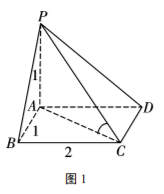$\text{A.}$ $\frac{1}{2}$ $\text{B.}$ $1$ $\text{C.}$ $\frac{\sqrt{5}}{5}$ $\text{D.}$ $\frac{\sqrt{6}}{6}$

C

#### 解析：①点击 首页查看更多试卷和试题 , 点击查看 本题所在试卷